# CS5483 week7 Lecture Tutorial Zoom

x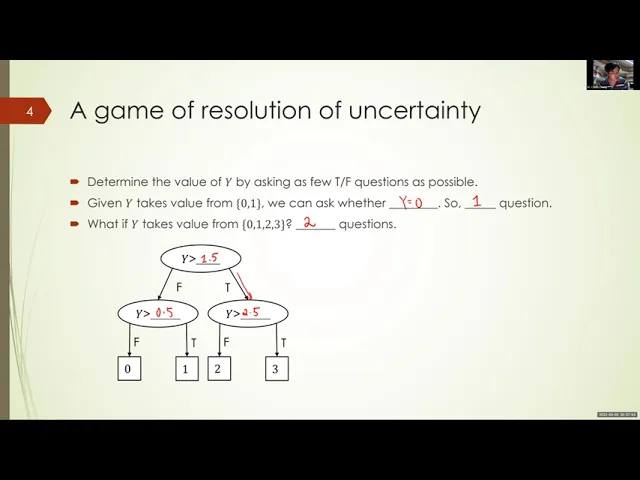Main point
Full script

Okay, let's get started for the lecture. I hope everyone has done a good job indeed. I can see your scores and the average is 77 something, so, which is pretty good, but i will still have to take some time to moderate your answers to see if there's any grading issues there, and i hope i can release the quiz result tomorrow or sometime before saturday. Okay so, yes, so, but from what i have seen so far, everyone have done a good job. So if you have any comments about how i should organize the online quiz better, let me know. It's quite likely that the exam would also be organized in pretty much the similar way for online remote exam. But yes, we'll see. Okay, so now for the lecture. Today i'm going to talk about something that i am most familiar with, that is, information theory. Indeed, i i must say that my research is in the area of information theory. Now, the lecture notes for today will be under week seven, and that is this information theory. So you can open this one, okay, okay, just to check if everyone can hear me.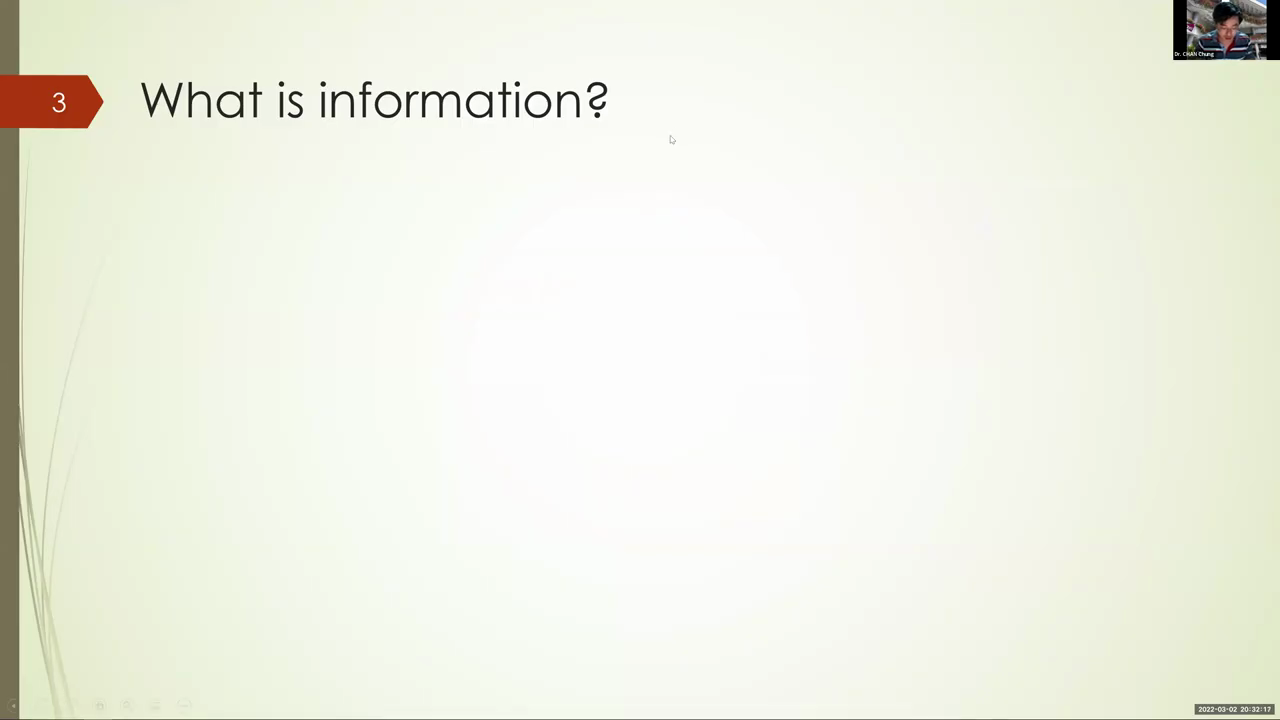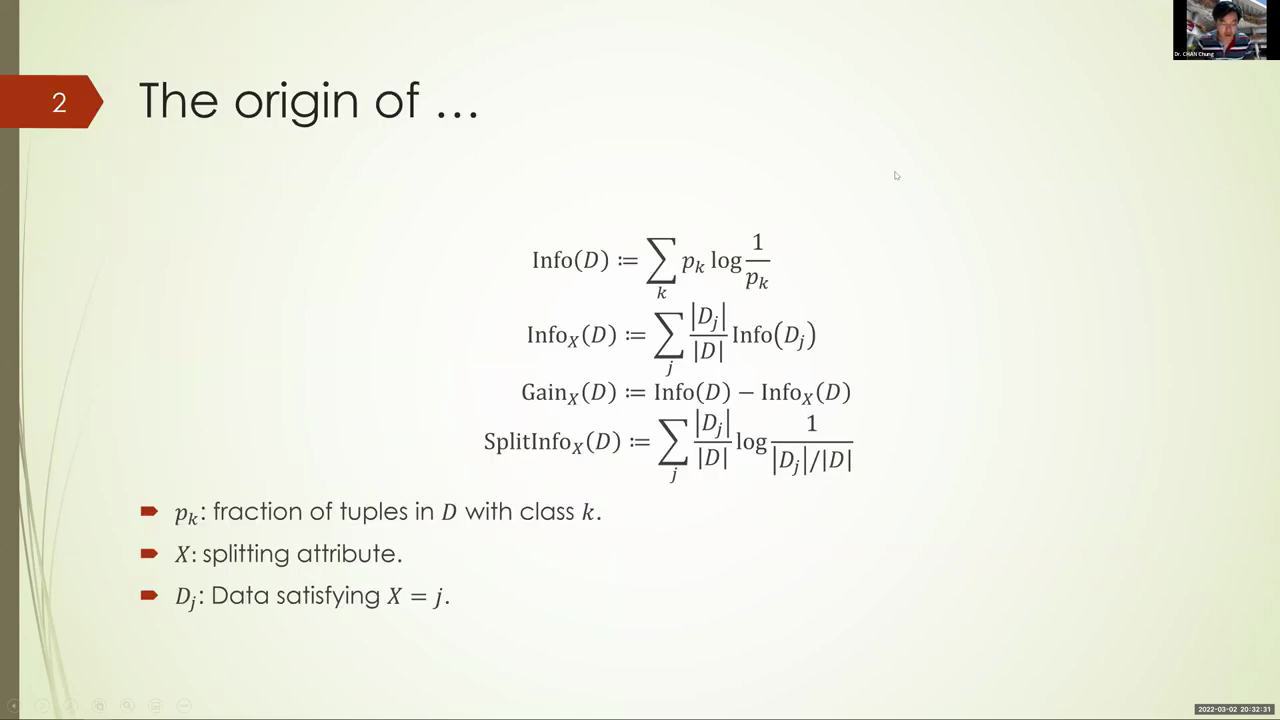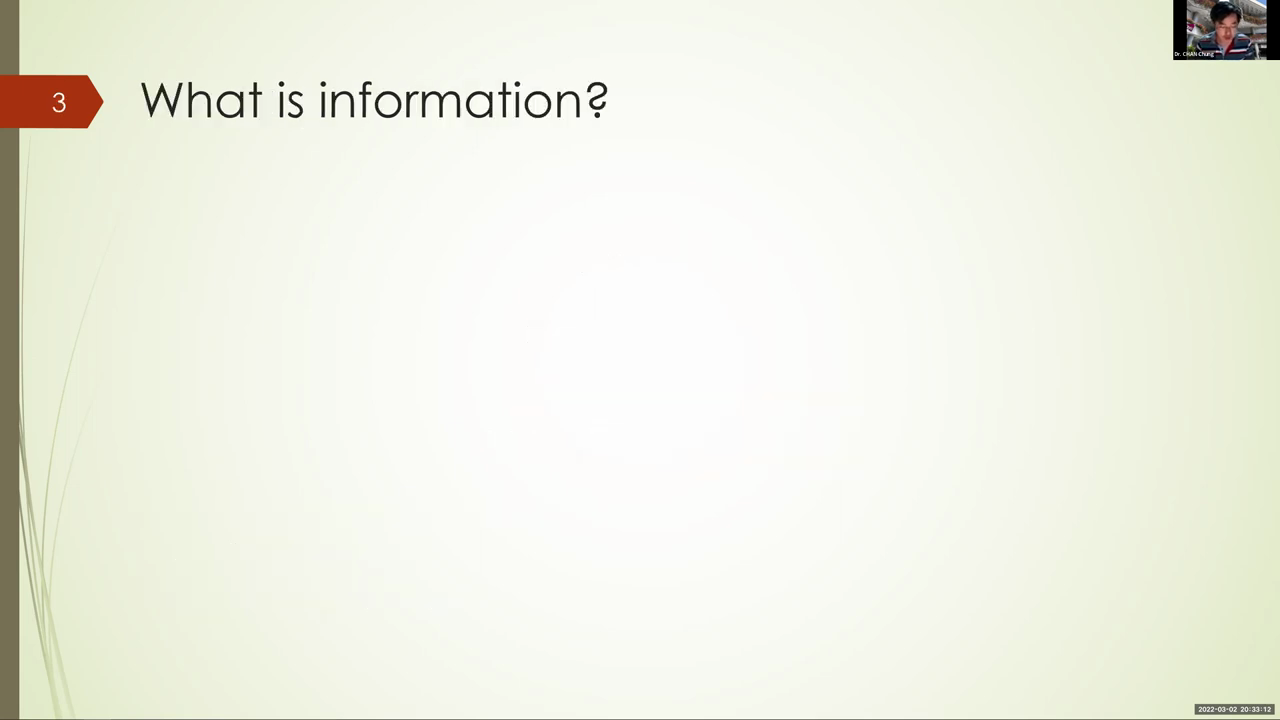Main point
Full script

If so, send me a chat message. Ah, great, okay, so what is information theory? So, again, let's motivate the idea. Well, what do we want to know about information theory. We want to know enough for data science in particular. We have learned how to build a decision tree and in the quiz you probably have also calculated the following quantities: the info of d, info x of t, the information gain of d, the speed information of d. I have taught you the formula and also give you some rough idea. Today i'm going to give you precise mathematical meaning, and that is from the theory of information. Okay, so what is it? What is information? Can we put a definition to information? Okay, and here we go.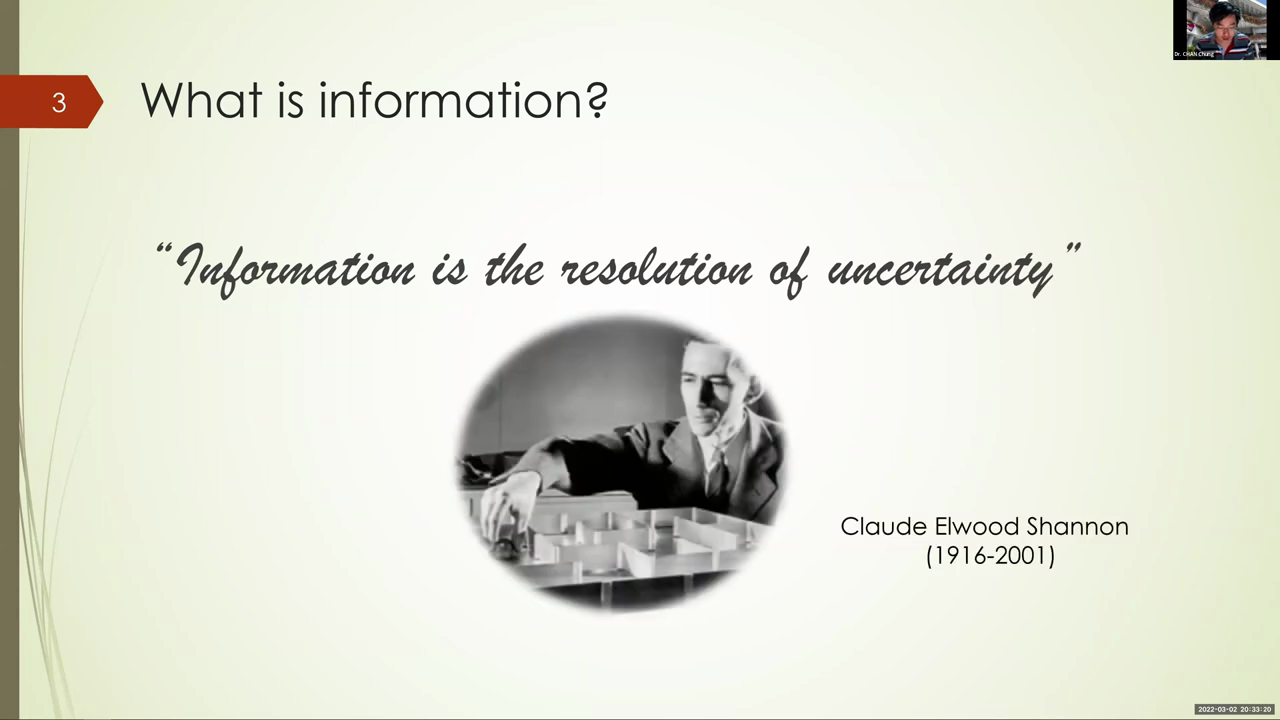Main point
Full script

That's the definition: information is the resolution of uncertainty, and that is defined by the father of information, edward avery shannon. Okay, and this is done. Well, half a decade ago, around the second world war. Now, what does it mean by resolution of uncertainty? I mean, pray, everyone, have a rough idea of what information is. Do you agree with this definition? Is it useful? Okay, let's play a game to understand this definition better, and this game is the resolution of uncertainty game.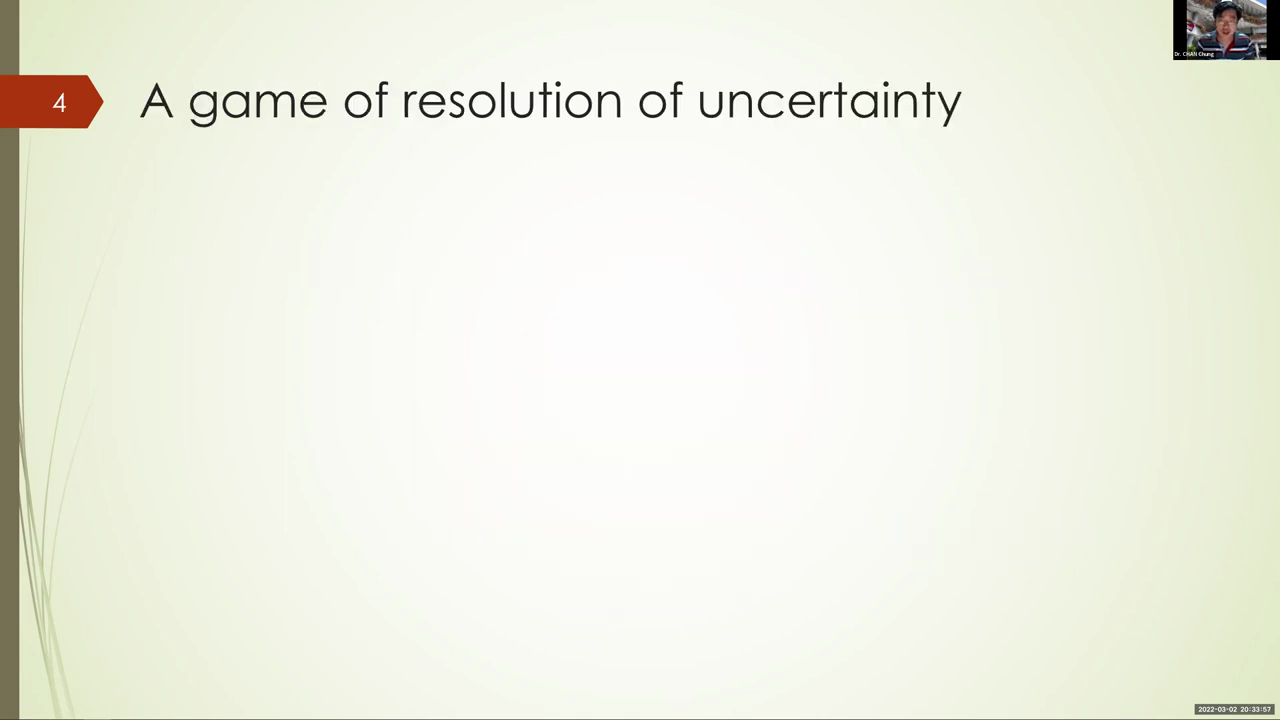Main point
Full script

Okay, how do you play it? Well, what i'm going to do is i will have a random variable, y, that is not shown to you. Now you have to ask questions to to guess the value of y. You can ask me any questions, and but then those questions has to be just true, false question. Okay, i will answer you, true or false, and based off my answer, you might be able to guess the value of y. Okay, now the the point of this, this game, is you want to ask as few questions as possible. Imagine every single question you ask cost you some money. Play you don't want to lose a lot of money. So how do you ask the questions?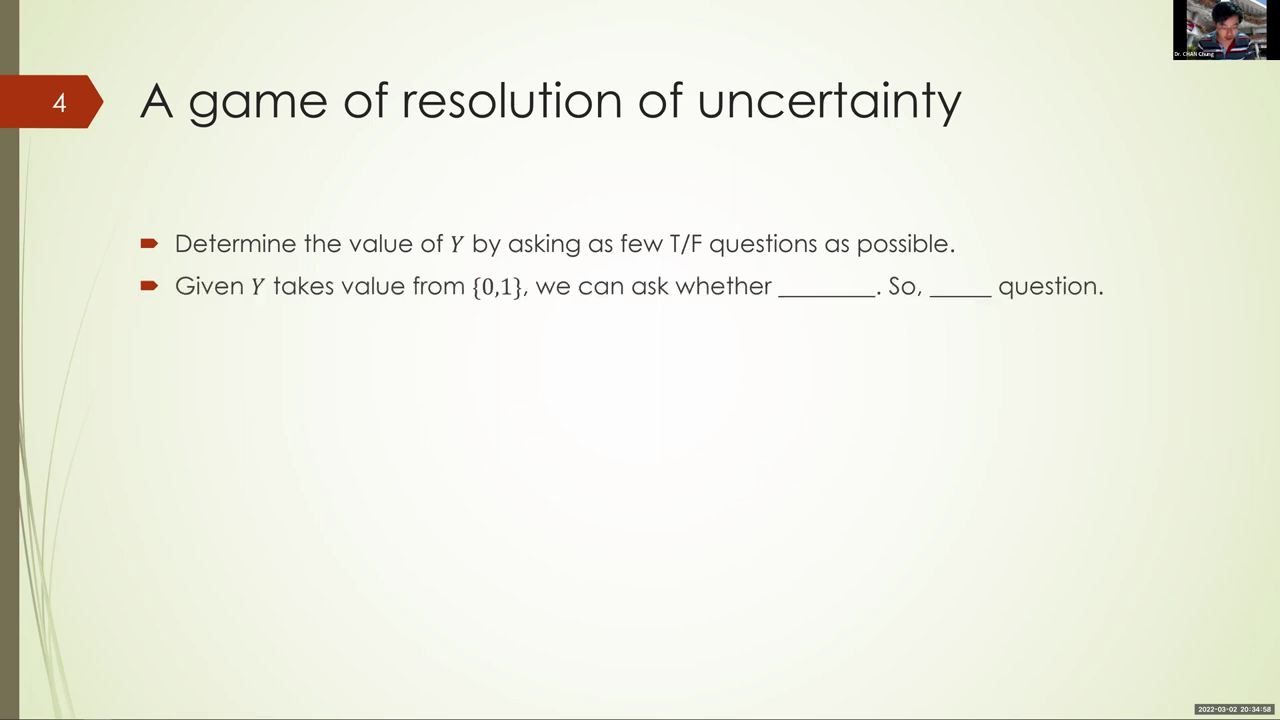Main point
Full script

Suppose the value of y must be binary, just like a binary classification problem. Okay, y can take value 0 or 1.. Now, how many true, false questions can you ask to be able to guess the value of y correctly? One, and what would you ask? Is y equal to zero? Okay, we can ask whether y is equal to zero, and if my answer is yes, then the value of y is equal to zero, right. If the answer is false, okay, then the value is equal to one. Okay, just one question is enough. And can anyone win? I mean, be able to guess, resolve the uncertainty of y with zero question? I guess not, because, well, you don't know whether y is zero or one. Okay, so what about the case when y takes values from zero, one, two, three. I mean there are four possible cases. Now how many questions do we need to ask? Two, two questions. And how are we going to ask these two questions? What is the first question to ask and what about the second question?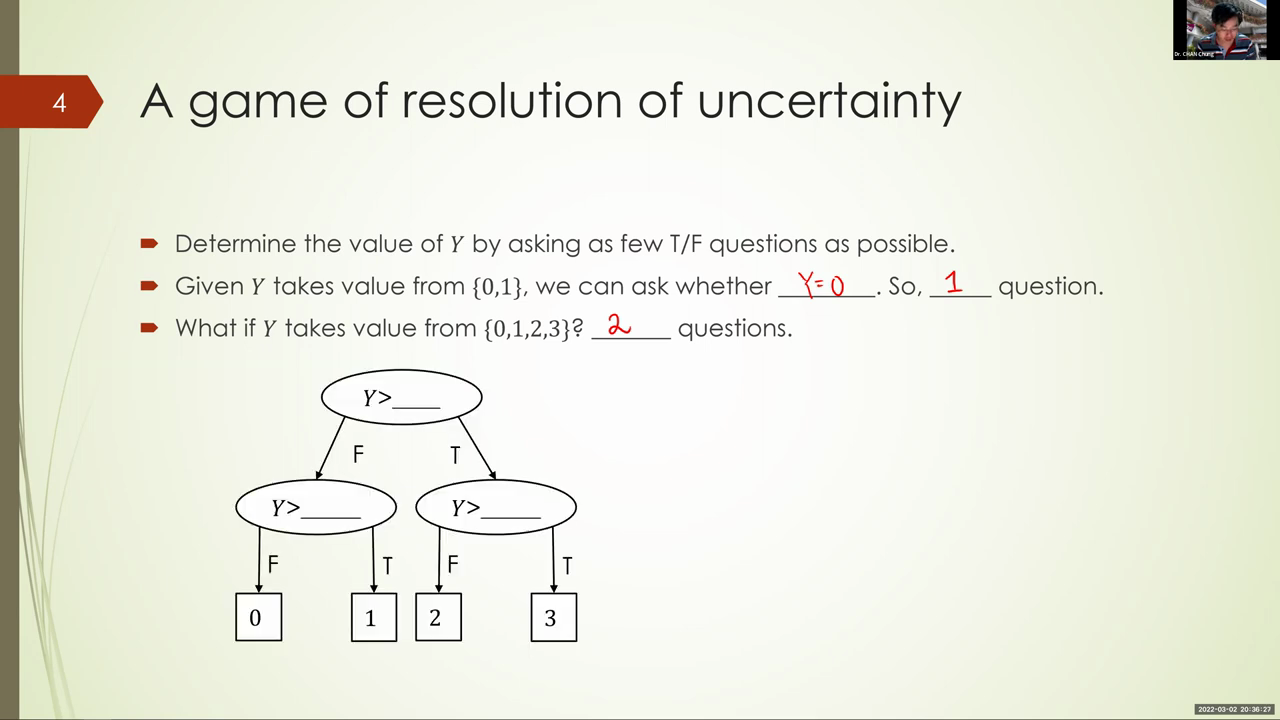Main point
Full script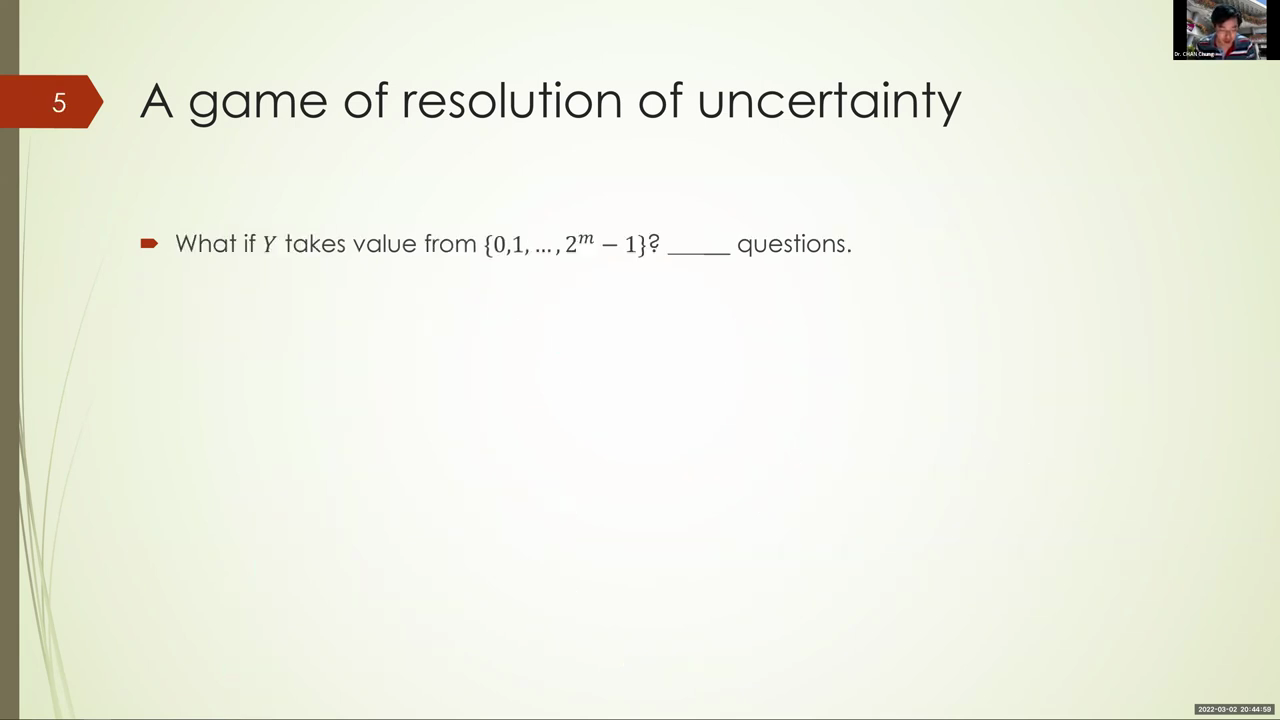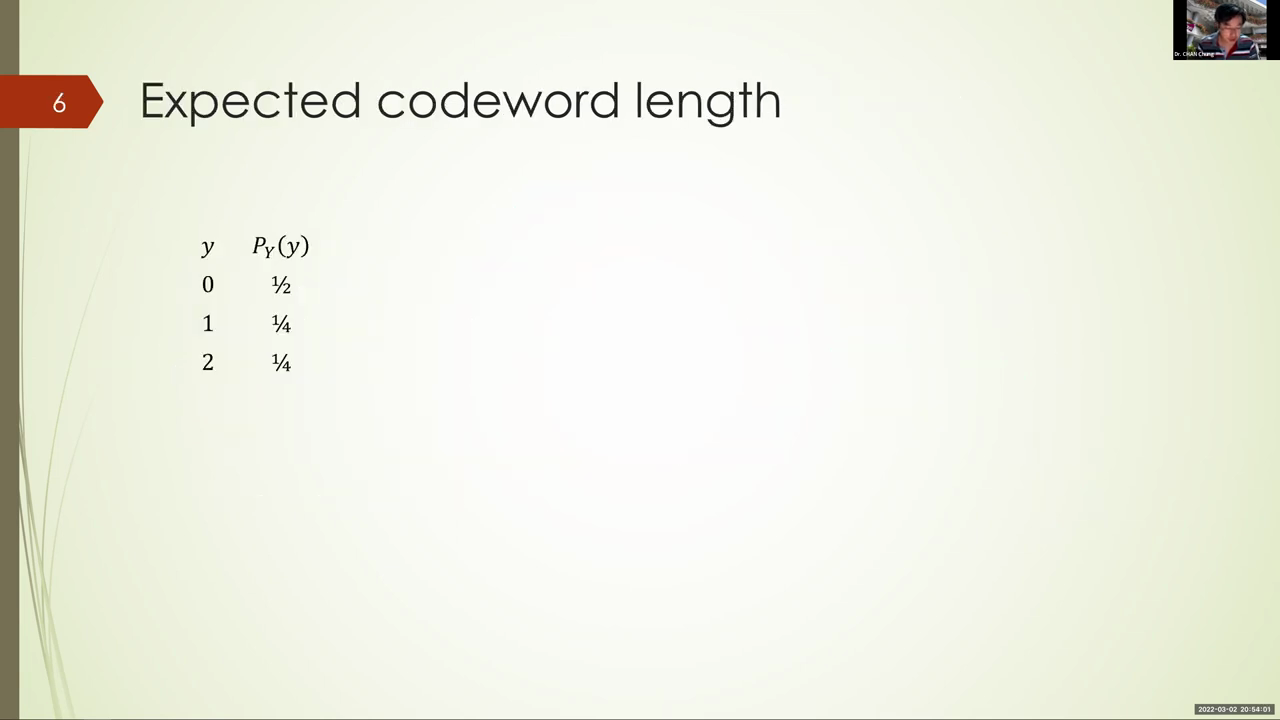Main point
Full script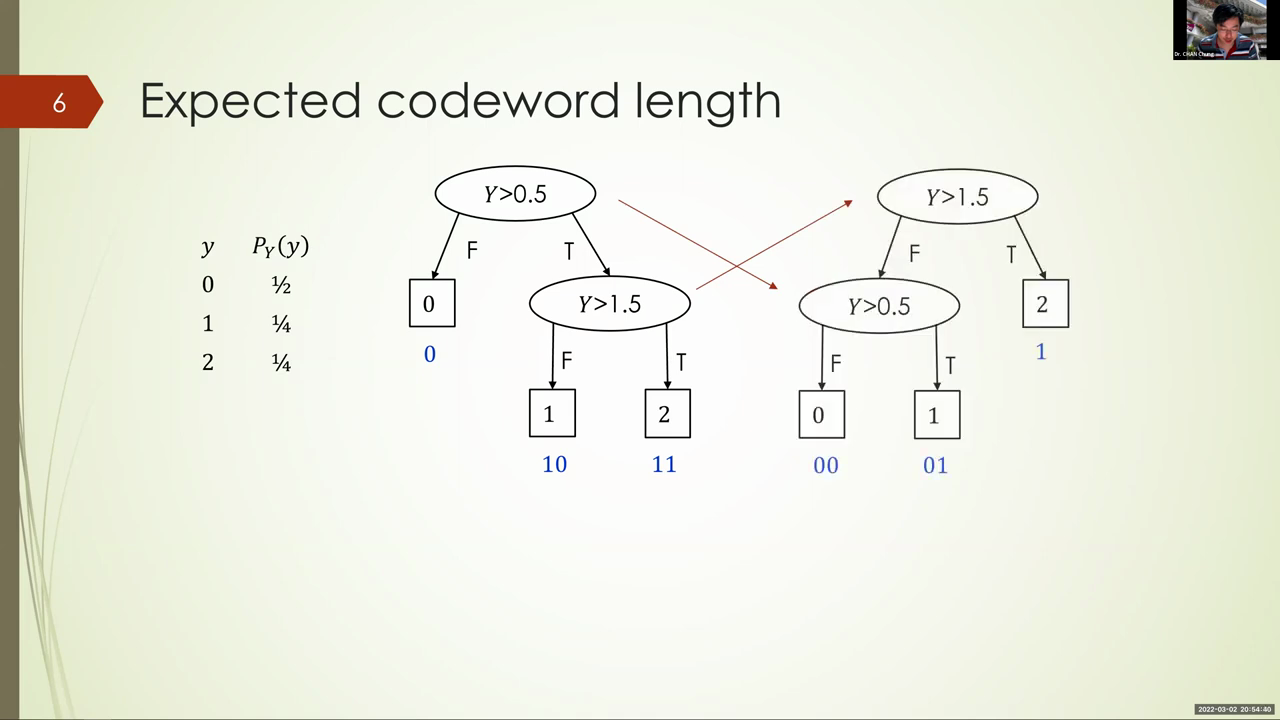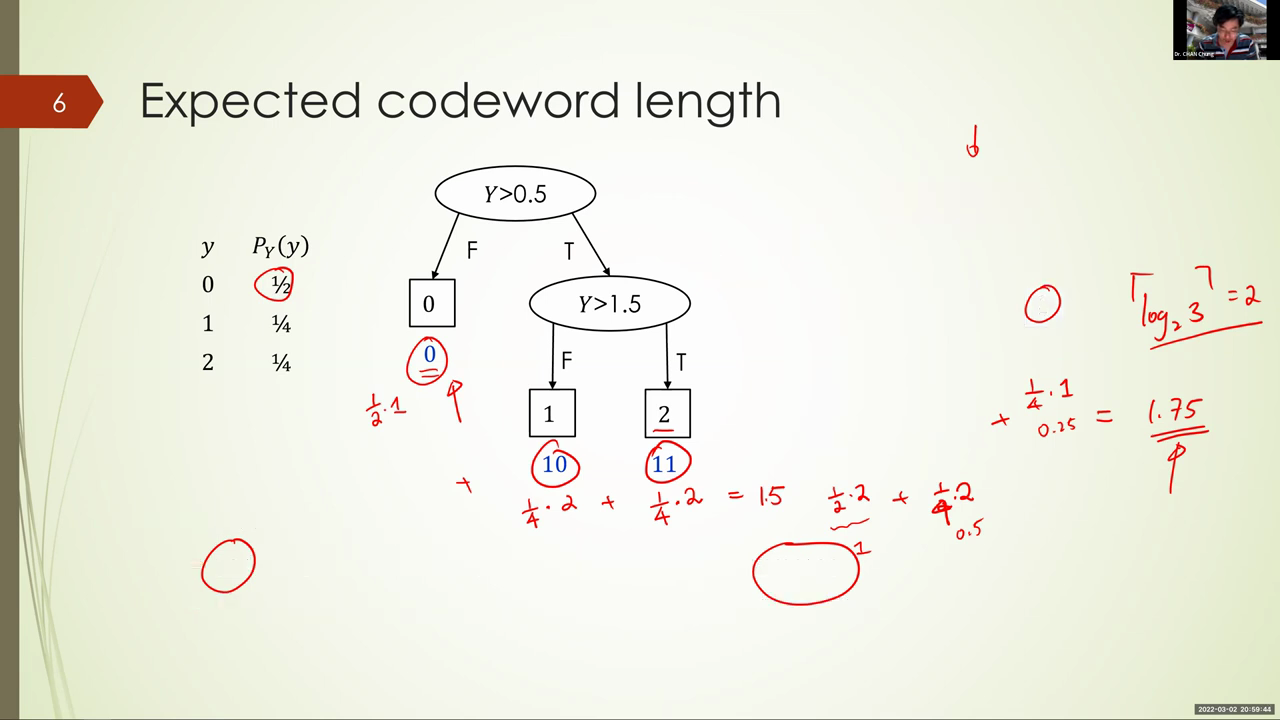Main point
Full script

So i mean coating trees. Okay, so the splitting point are very similar and indeed they are exactly the same. I mean, it's just the order of split. So in the first tree, i ask, okay, whether y is bigger than 0.5 first. If it is indeed smaller, okay, then i know for sure that the value of y is equal to zero. But if it is bigger, then i still have two possibilities. Well, y may be one, one, maybe two. How do i resolve that uncertainty? Well, i can ask again whether y is bigger than 1.5. If y is bigger than 1.5, i know for sure it's two, and i mean the other way, i know for sure it is one. Okay, so this is a valid tree, and if i translate it to code, then i would have this variable length code. Well, because for encoding zero i only need one bit, but then for encoding the other two values, one and two, i need two bits. Okay, now compare this with the tree on the right hand side. The only difference is i swapped the order of the two questions. I now ask whether y is bigger than 1.5. First. If it is bigger, then i know for sure that the value of y is equal to 2.. Otherwise, well, there are two possibilities. It may be zero, it might be one. I i will ask the other questions: is y bigger than 0.5? Then i will be able to resolve the uncertainty. Okay, which tree is better? Okay, do they give you- well, log base two of three questions about sitting on that? That is equal to two. Well, i still need to ask two questions for each tree, but notice that sometimes i can get away with just one pressure. For the left hand side, if the value of y is equal to zero, i just need one question, e on the. On the other hand, on the right hand side, if the value of y is equal to one, i need one question. But if y is equal to zero, i actually need two questions. So it's slightly different because of the cases. The question is which one is better? Well, better, let's say, in terms of the expected number of questions, i need to ask or expect, the codeword length. Okay, can anyone calculate the expected codeword length of the left hand side, the left tree and also on the right tree, and then compare to see whether they perform the same or they one is better? Okay, which encoding is better? Is it the left one or is it the right one? So, on the left hand side: well, half of the time i get zero. So half of the time when i calculate the expected convert length, what i would do is: well, half of the time the code length is equal to 1, but then a quarter of the time the codeword length is equal to 2, and also a quarter of the time the codeword length is equal to 2, and then i sthem up, i get a expected codeword length. And what is that? 1.5, very good. Now what about the tree on the right hand side? I have one half times two, one half times, sorry, one quarter, a quarter times two and then a quarter times one. And what do i get when i sthem up? Okay, so i get. So i have one here, i have well, 0.5 here and i have 0.25 there. Yes, 1.75, notice, this is longer, meaning it is well, no good. So the left tree is better, and because the expected codeword length is smaller. So i hope the idea is very clear to you. If you know the distribution of the symbols, then you can calculate something called expected codeword length. And the expected codeword length is indeed smaller than log base two or three. Okay, well, for the best tree, but for the tree on the right hand side it is actually bigger. Okay, so we can actually do better. So well, remember us that's log base 2 of 3 is 1.58, but then what we can achieve is the better one is 1.5. Okay, so we do better. Okay, in general, how do we encode?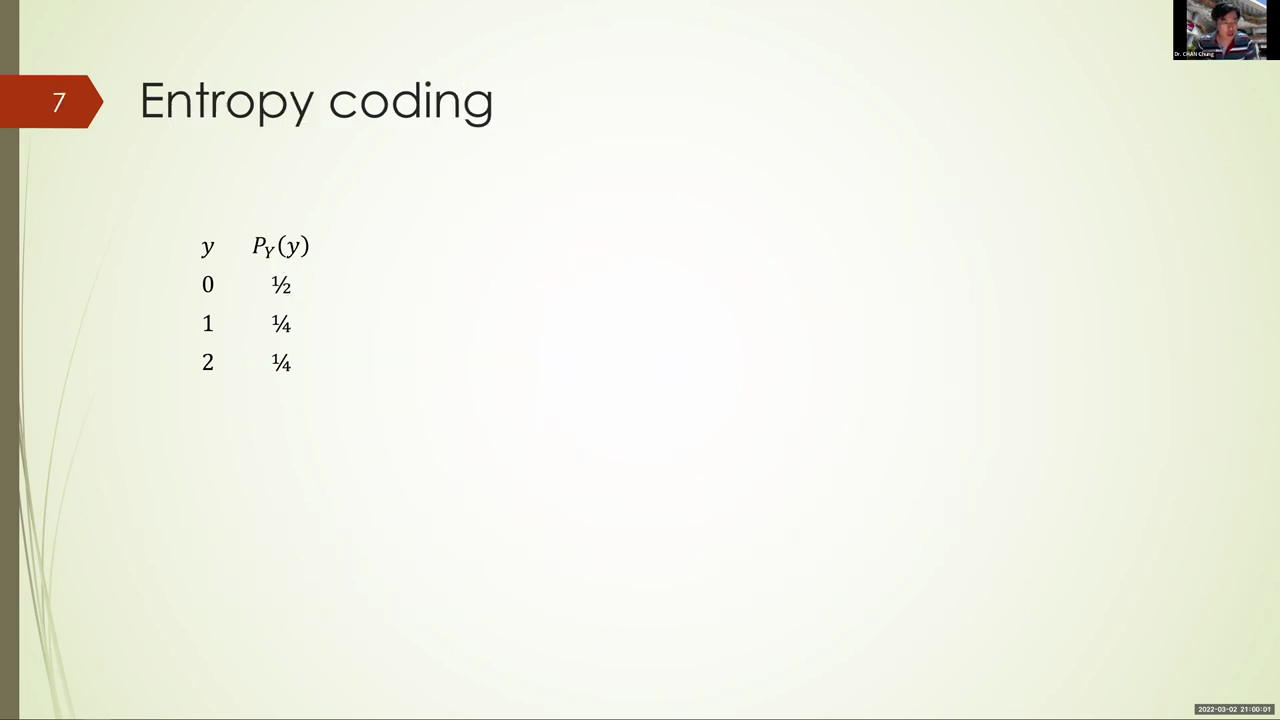Main point
Full script

How do we encode in such a way that we have very small expected codeword length? I mean, do i have to try out all the possible coding tree. If so, that would be very time consuming. Okay, there's something called entropy coding, and by the name of this coding strategy you already can associate it with an information quantity. I'm going to talk about that is entropy. Well, i pray. Just briefly mention about entropy before, and i defined it well, same as info of d. Well, indeed, the entropy is a notion in information theory, and when you apply it to data science to evaluate the impurity of a data set, then you get another name that is called the info of a particular data set, d. Now. So what is entropy and what is entropy coding? So let's see. Okay, so let's, let me use some mathematical notation now to denote the codeword length of a particular value, y, to be l of y. Okay, okay then entropy coding says that we can have a variable length code to encode the value of y, with the length of the symbol y equal to the setting of the log of the reciprocal of its probability. So let's calculate the reciprocal of the probability and then the log of that and the ceiling of that. Well, to on on this particular example. Okay then. Well, when i take the reciprocal of the probability, one half becomes two. So this is just log of two. Again, unlike the equals interface where log corresponds to the natural logarithm, in all my natural log correspond to log base 2. this is equal to 1. Okay, that means i'm going to assign one bit to the simple zero. Now the second, the next one, okay. Log of four. That is two. Okay, and log of 4 again, so 2.. So this x actually corresponds exactly to the up to the better coding tree we just identified.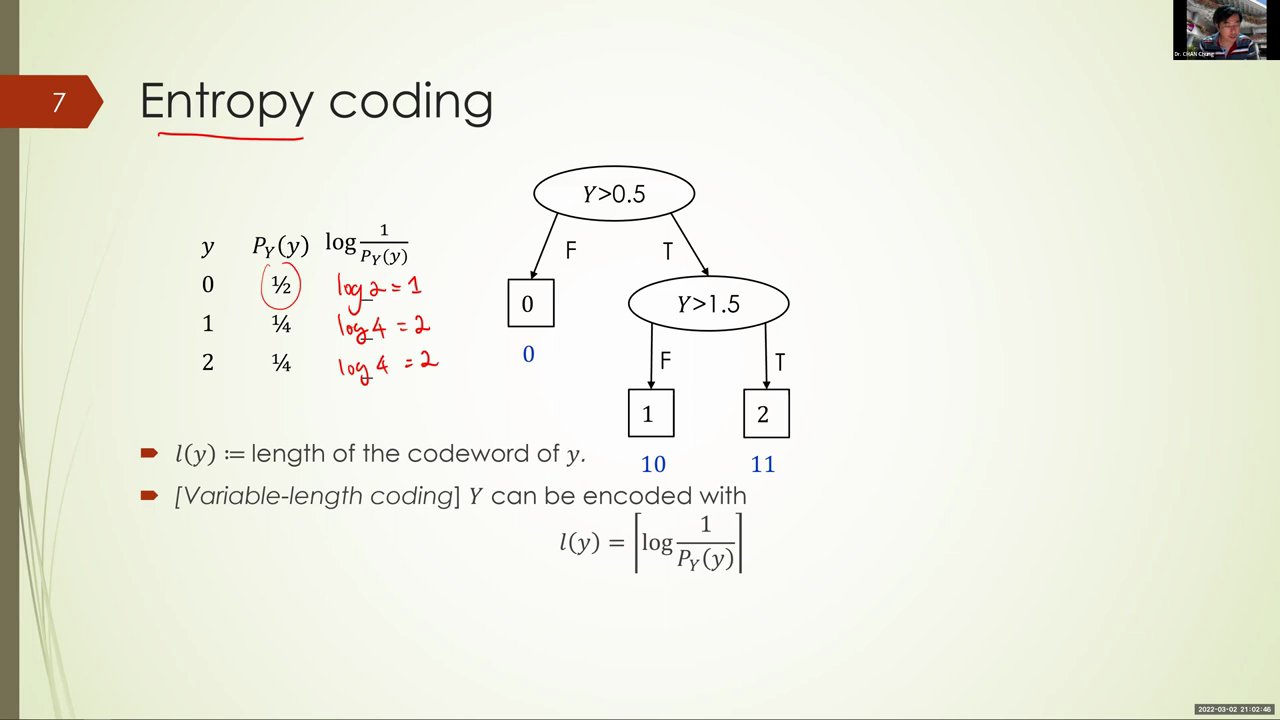Main point
Full script

So for this example, the code links i used to encode a more probable symbol is shorter because i take the reciprocal of the probability before i take the log. So log is an increasing function of this argument and the reciprocal, of course, is the decreasing function. Then overall we have a decreasing function with respect to the probability. So the larger the probability, the the more probable a symbol is going to be observed. We actually assign it with a shorter code. That makes perfect sense because that is going to reduce the expected codeword length. More probable code words receive less bits to encode. Okay, so the expected codeword length is exactly equal to what is that? If i use entropy coding, what is the expected codeword length? But that would be well. First of all, i would write p of y, of y, times l of y and then sover all the possible values of y. Very good, that is, and what would that be? So so this is, of course, one notation is expectation of p of y and capital y. Now, when i write this expectation, notice that i use the capital value y to denote the random variable y and i use little y to to exp, to to for its realization. So this, this notation will will be clear, so that other people know what is actually random and what kind of a randomness we are trying to take average off now when. So actually sorry, i shouldn't write here right here, my mistake should be l of one, okay, and that is info of the data d. Well, we don't have data here. We have the class distribution precisely specified here. But then we also have something weird, because it is, let's see, like there is a ceiling there. Okay, so expected codeword length is almost like the entropy, because if i, if i substitute this as log of 1 over p, y of y, that is exactly the definition of entropy of y, but then i have a ceiling there. Okay, in this case, the expected codeword length is precisely 1.5 and there is no. I mean, the ceiling is exactly the log of reciprocal itself. I mean, because all these are integers. So this is exactly the entropy in this example.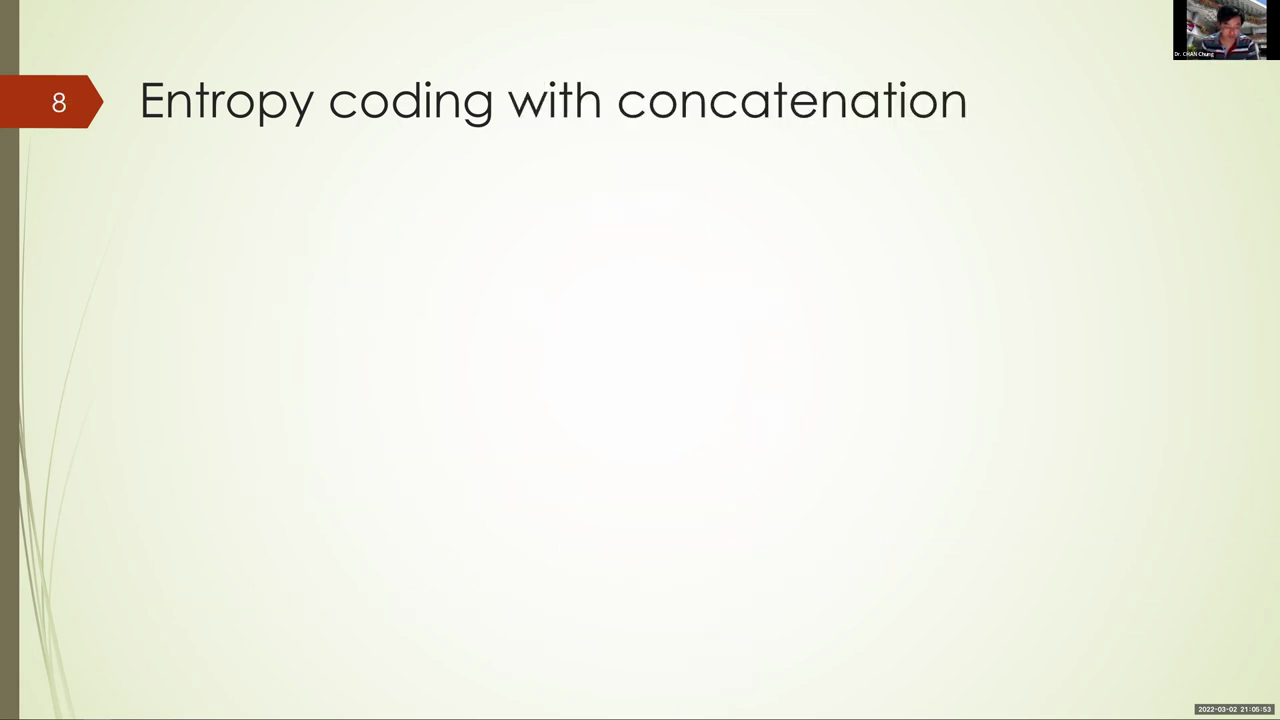Main point
Full script

Okay, now, how can i well say, indeed, that the expectation is precisely entropy? Well, i have to have some way of getting rid of the setting, the trick we have already applied before, i mean just now, when we insist to say that i mean log base, two of three questions is possible. We can ask that many questions. The strategy we use is concatenation, right. So if i can con, well, if i concatenate this, a sequence of symbols, and try to find the expected codeword length of this sequence, maybe it will get exactly to the entropy. I mean, you can probably just, i mean, prove it in your head. But indeed i'm going to prove something even more. I would say that expected coding rate is almost surely the entropy. Okay, so let's calculate, i mean let's prove. One is just that formally so, the now, according to entropy coding, all we need to do is to assign the codeword length to the sequence by the ceiling of the log reciprocal of the probability. Okay, so that is exactly what entropy coding is, but i just applied it not just to one symbol, but to a sequence of symbols. Okay, now what i'm going to do, i'm going to simplify this expression so that i can prove what i just said. Almost surely, the expected- well, actually the cookware length, not the expected one, the codeword length, almost surely is equal to the entropy. So how do i do that? Well, i can, because all the y's are independent and identically distributed. I can rewrite the joint probability of this sequence as a product of individual marginal probability. Okay, and this is by the assumption of independence and also identical distribution. Now, once i do this, what can i do? Well, we often apply the trig log of a times b is equal to log a plus log b, and i can apply that here as well to further simplify things. And that will give me the s of the log reciprocal of individual probability of the marginals, summed over the the symbols. I, okay, okay, so i get a s. Now to be clear what i'm going to do again, to apply this derivation directly to the example we have seen just now.Main point
Full script

That is the case when y1 and y2- they are iid, with the probability zero, equal to one half, and probability of 1, 2 equal to 0.25. Now i can calculate, well, the joint probability. So this joint probability p of y1- y2 is just the product of py- y1 and p, y, y, two. Now, for y one equal to zero, then the probability is point five. When y one, y two is equal to one, then the probability is point two, five. Now i deliberately write it so that i put the code length in the exponent, okay, and when i then combine them, the code length needed to encode zero one is indeed three bits. So this you can actually see it here, and when i take the log of the reciprocal, i get three. So now you get a list of codeword lengths and you can then divide it by n and see whether it reached the expected. We reached the entropy of 1.5. If you sthem and divide it by. If you sthem and divide it by the number of symbols- two, okay, then what do you get? So if i sthem- and well, sorry, i shouldn't say i s sover- the disposable cases and and i, what i would like to do is we have where we observe two, three and so forth, each of them, i'm going to divide it by n, okay, and i would like to. Well, you can see that it is going to be random. If i divide it by 2, you will get 1, 1.5, 1.5, 1.5, 2, 2, and then 1.5, 2 and 2.. 1.5 appear quite often and indeed, what i'm going to say is that when n goes to infinity, almost surely you will all well see 1.5. So that is, with probability equal to 1, you will be seeing a value very close to 1.5. Okay, and what do i mean? Again, i'm going to apply the same trick as before. I will just say that ceiling only contribute order one to the expression. But then when i divide it by n, then the order is order one over n, which will actually goes away as n goes to infinity. So, as n goes to infinity, then we are looking at just this expression there. What is this expression? And why do i say that? Almost surely it is the entropy. Well, that is by the law of large number. But the law of large number, this is a sample average. Well, average of what average? Of log reciprocal, of the probability. Now you are, what i'm thinking is i'm thinking of the log reciprocal as a random variable. And what is random about this? Well, it is the argument y here that is random. The value of the symbol is random, okay, and i'm using attribute coding formula, which is basically the ceiling of the log of the reciprocal. Now, when i look at this expression, this is nothing but the sample average of iid realization of this random variable log, one over p, y, and, by the law of large number, sample average converge to the true expectation, almost surely, with probability going to 1 as n goes to infinity. And and what is this expectation? This expectation is exactly the entropy of y. Now i can give a very precise operational meaning of entropy of y. It is indeed the covert length you need to encode the random variable y. Therefore, it is just the information content, okay, so so i hope this idea is clear. But then the question, of course, is: is entropy coding the best possible? I mean, can we do better than entropy coding? Entropy coding is an achievable scheme. Well, for the ring, by achieving film is a particular scheme that can give you a corporate length of entropy of y. It doesn't say that it cannot give you better. It is possible that you might come up with a coding scheme that is even better than attribute coding. Is it possible? Well, no, the answer. The answer turns out to be no. Indeed, you can prove, using graphs, inequality that you can never encode with the codeword length smaller than the entropy of y, and this is what we mean by a converse result in information theory. Converse means a result that can prove that information theoretical limit that applies to any coding scheme. What is very elegant about information theory is we somehow can give you the precise limit as to how much you can do. You can ask: okay, if i have a file, then what is the minim, what is the minimfile size? I can compress that file too, and that is what information theory, like the converse result, can tell you. Indeed, entropy of y is exactly that quantity. If you think of y as a file you want to compress, then entropy of y is the minimsize of that, what, what that file can be compressed to. Now, speaking about this conversion result, i mean the argument is quite convoluted, but you can do it. Basically, there's some thing called a craft inequality saying that, no matter what coding scheme you use, if you require the coding scheme to resolve the uncertainty of y, then it must satisfy the well, the, the inequality that this is. This is less than equal to one, where l i correspond to the length of the code you assign to symbol i. Okay, now, once you have this inequality, then you can think of it as a constraint optimization problem. You try to minimize the expected covert length, which is also a function of li. You try to minimize that subject to the constraint here. Well then, you can apply some well relaxation, think of l i as not potentially a real number, not a integer, and then you solve that problem. You find that indeed, the solution is, the optimal solution of l i is exactly, according to entropy codec, this log of one over the probability, and that gives you entropy, and that is the minimpossible, okay. So i've told you the proof idea, but i actually haven't told you the proof. It's a bit too heavy, it's not required for for this course, okay, but it is important to understand the interpretation of entropy, the operational meaning of entropy.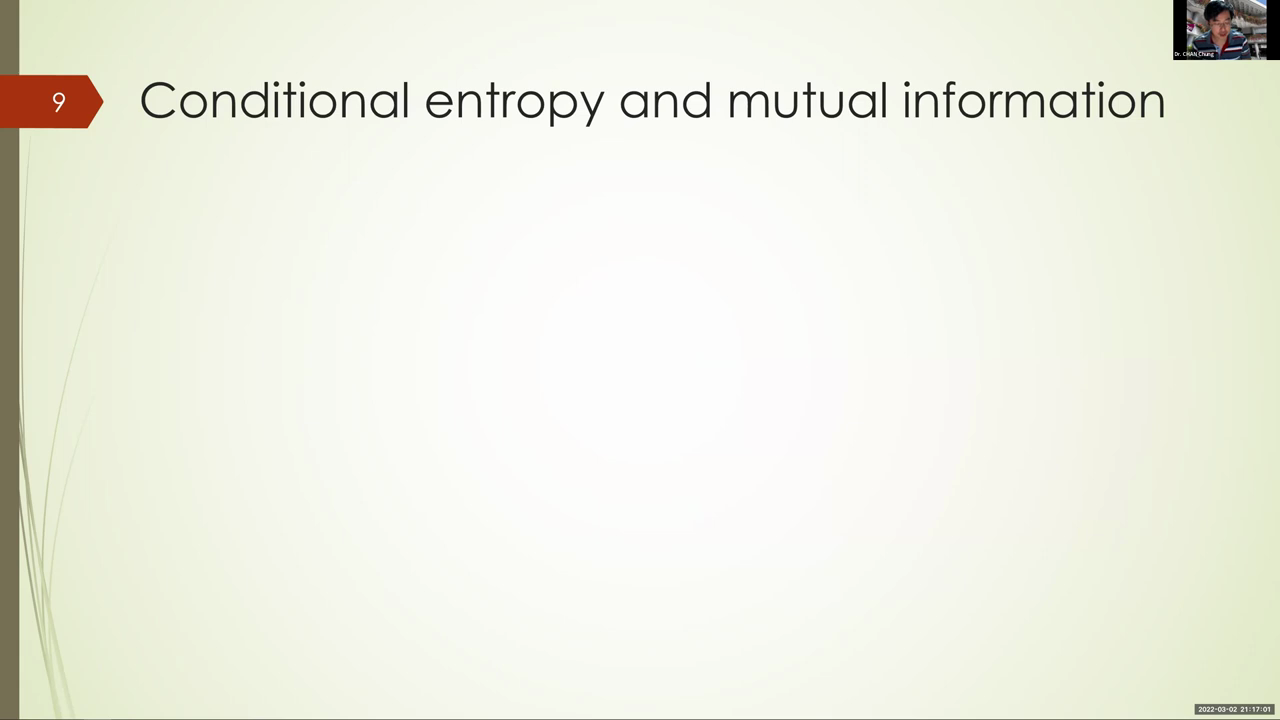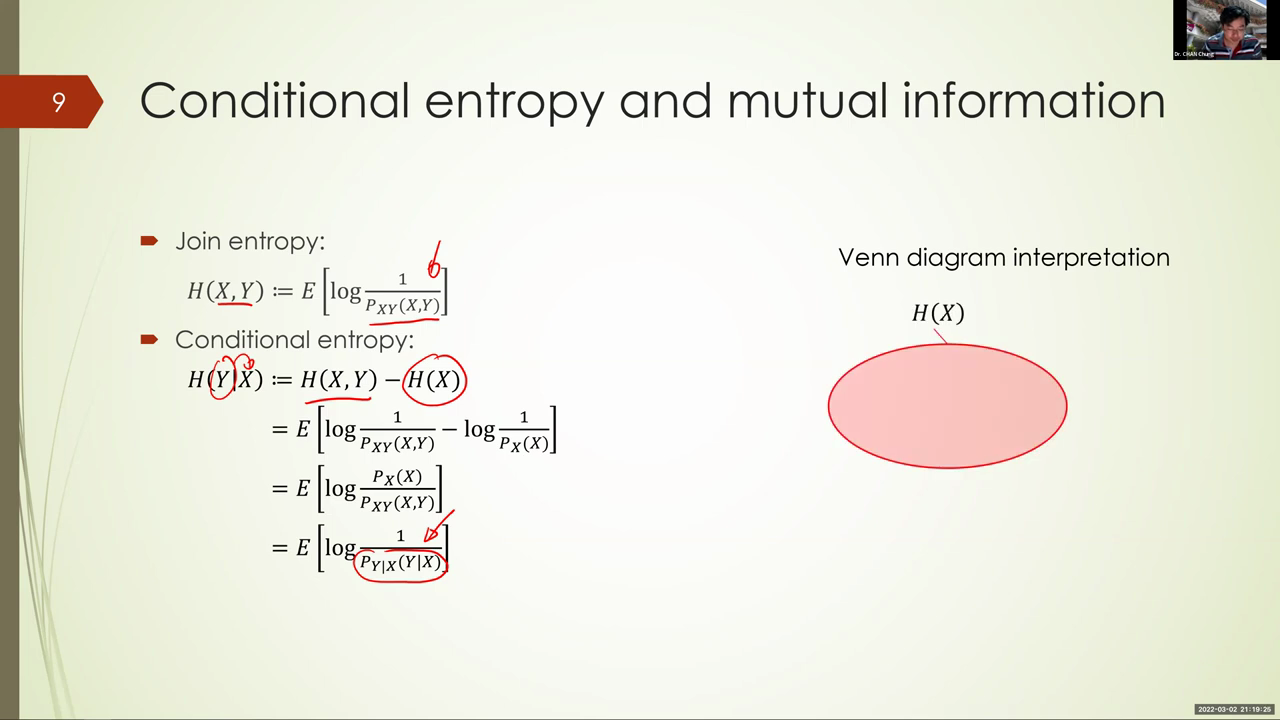Main point
Full script

Now then, okay, we can also talk about entropy not just for one random variable, but a concatenation of random variables, and this vendor would need not be iid. So all we need to do is to: we just think of the, the random variable, as a random tuple or random vector, okay, and we just apply the same formula to measure the information content of that. So instead of using just the probability of x over really a y, we use the joint probability and we can also define something called the conditional entropy, and the definition is this: so basically, you look at the entropy of x, y and subtract the randomness of x. What is trying to say is, what is the information content in, why that is not in x? Well, when i look at the joint entropy, i'm looking at the information content of both x and y, and when i remove the information content of x, then that is exactly what defines a conditional entropy, that is, the information content in y but not in x. Okay, you can apply the linearity of expectation and you basically can simplify the formula of entropy to the formula for conditional entropy. Here i apply the base rule to d to rewrite the, the ratio of the probability, into the conditional probability. So in the formula is actually quite easy to remember the conditional. Well, entropy of y, given x, is nothing. But you take the expectation of the log reciprocal probability, but that probability is the conditional probability, that is the probability of y, given. You have observed x. Now joint distribution is in the similar way. We modify the original entropy formula, i mean we replace the probability of x by probability of x and y. Okay, so in the same way. Indeed, a better way to remember all that is to use something called the rand diagram interpretation. Now, what i'm going to do is to think of the information as a set, a set with the area that is proportional to the information content. If you have more content, then the set become bigger. Okay, now the.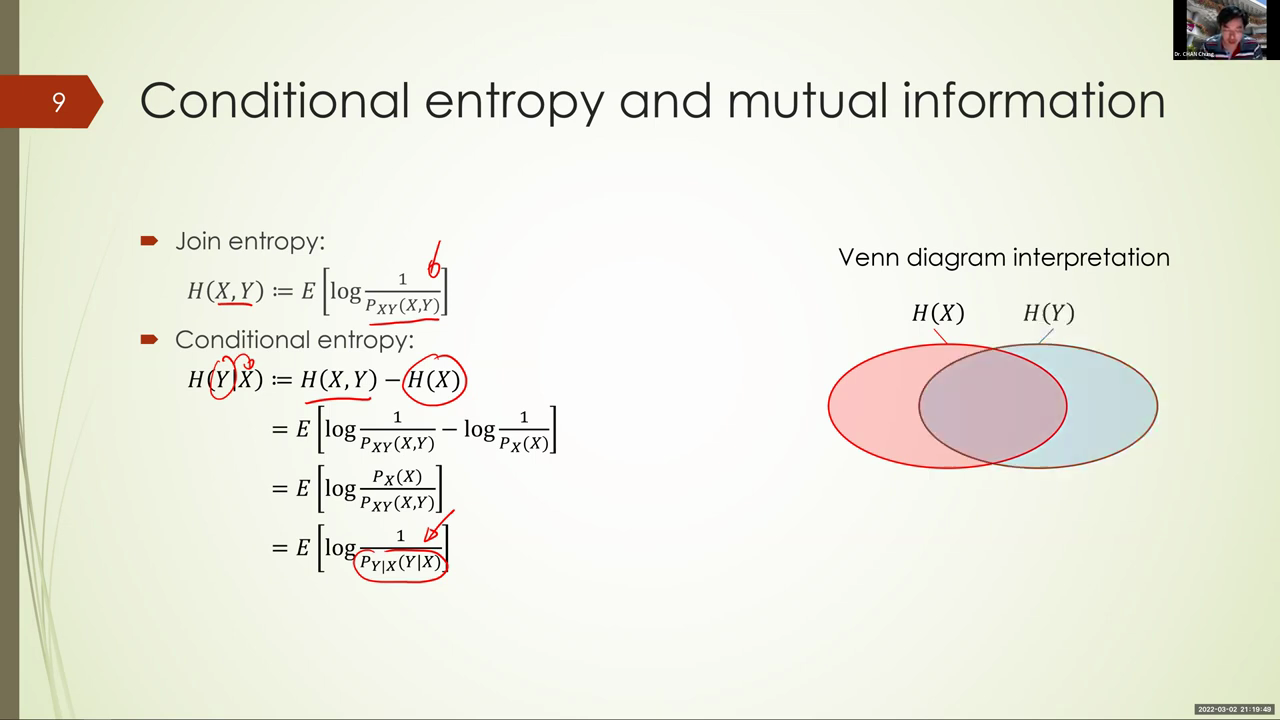Main point
Full script

The information content of x and y may overlap. Maybe two files actually contain the same content. Okay, maybe you are talking about, let's say, bible. Okay, bible can be written in english, can be written in chinese, and, but essentially they have the same content. So if you draw it, then they will have a lot of overlap. Okay, now, the joint entropy is the is the area of the union. Okay, now, if you have to well, describe the moon shaped area, a, what would you describe? I mean, how would you describe it in terms of entropy? Can anyone tell me the entropy value for this? Is it entropy of x? Well, no, because x actually is the area of the entire red part. What? What is it here? Well, the moon shaped area is the information content of x, is part of the information content of x, but that part has nothing to do with why? Because y is on the right-hand side. So, exactly, the area can be calculated as the entropy of x and y minus the entropy of y. Right, and what is that? Well, as defined on the left hand side, it is the conditional entropy of y given x. So to understand conditional entropy is very easy. You look at the venn diagram and you basically see that this area corresponds to region that is not contained in y. So then that is the information in next in in x but not y. So, sorry, i should say x given y, okay. Similarly, that is y given x there. Okay. Now what about the middle one? What is the middle one? Well, middle one, you can apply the formula, so the union, okay. So how do you get the middle one? How do you express them? The middle area, so i mean this one in terms of entropy. Can anyone write a formula? Well, i have, well, i have. Well, i can. Actually, very good, okay, if i take entropy of x- so this is the red part- and then subtract the moon shaped area, then i get this area right. Okay, very good. But of course there are other ways to calculate this as well. I can take the entropy of y, that is, i can. I can take the blue, blue shape there and then subtract this moon shaped area. That is also okay. Now, indeed, you can also write it like this: minus this: how come? Well, you can also write it like this: okay, how come?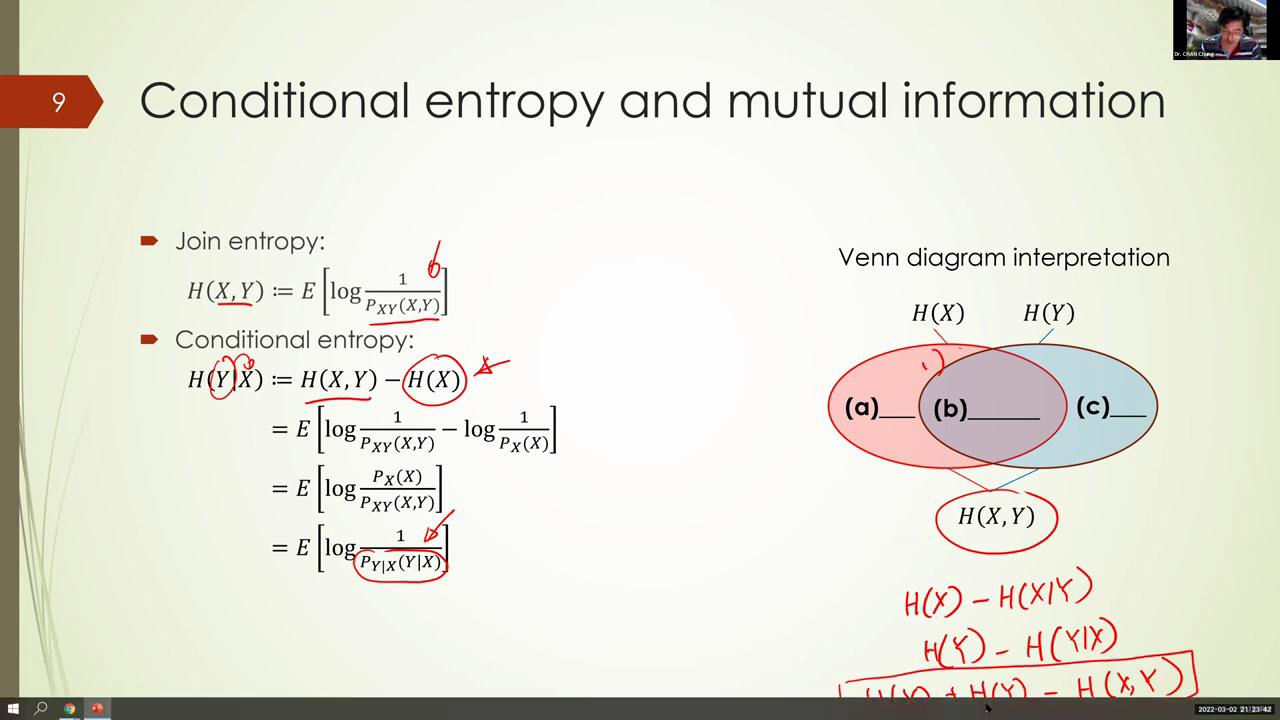Main point
Full script

Well, you are taking the area of x, area of y, then you subtract the area of the union. But when you add area of x and area of y, well, the middle part is double counted, because this median region appear in both x and y. If i just want to get the, the area of the middle region, i would have to submit: well, subtract, subtract the area of the union, and i'll get this. This is called the inclusion, exclusion principle in combinatorics: inclusion exclusion. Okay, but i think it is pretty easy for you to see, and indeed you can also apply the formula for calculating the conditional entropy of y given x to get this formula. How well, entropy of y given x is nothing but entropy of x, y minus entropy of x. And there you get this: entropy of x and h of y minus entropy of x, y. Okay, i hope this is clear. Okay, a. We call this area the mutual information between x and y. Why do we call it the mutual information? Well, just from the rand diagram. You know, this is the information that appear not only in x but also in y, so it is mutual. It is the information that is mutual to both x and y. That's why we call it the mutual information. Okay, and this quantity is extremely important because this is the quantity that you use to build a decision tree.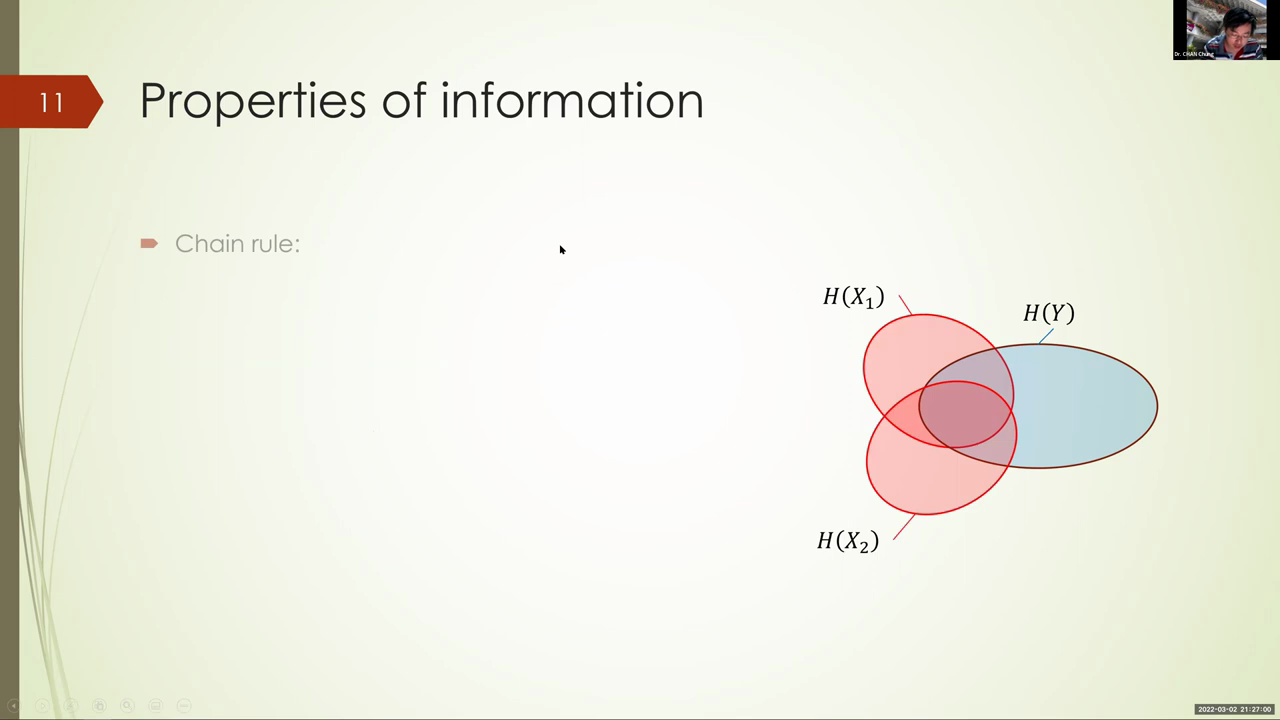Main point
Full script

Well, not quite well in the early version, what we call the like dichotomy three. Dichotom mean three, okay, so that's sorry dichotomizer. Three, okay, that use information gain. But then later on, we use information gain ratio. That is, the later on, j40 and c4.5 realized that there's a bias towards outcome, with many, many sorry features, with many, many outcome. And to normalize it back we need to use something called the speed information and all that. Now, actually, before i say all that, it would be a good idea for me to point out. Indeed, the formula that you have been using is exactly mutual information. So, first of all, you already know that info of dj is nothing but the entropy of the distribution of y. Well, dj is indeed the conditional, the conditional version. So it's the entropy of d when x takes a particular value of j. Okay, but this alone is not the conditional entropy of y different x. Okay, this is sorry.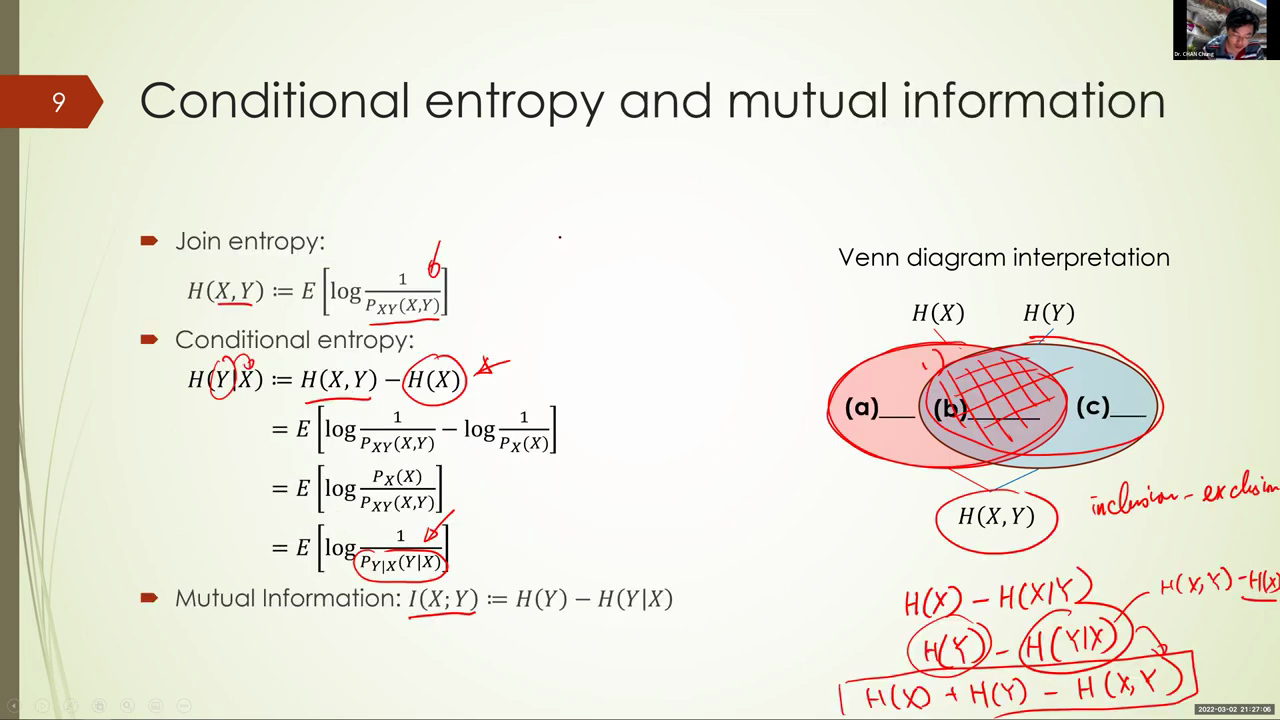Main point
Full script

So this alone is not the conditional entropy of y given x. X, we also have to average over the possible value of j. This, this is what we usually write it as- x equal to j. So this is a particular realization of x. But if we average it out for all possible outcome of x, then we get exactly this one. Notice that here x is a random variable which can take different values of j, and this averaging will give you the conditional entropy of y to the x. So info of x is nothing but conditional entropy of y given x. Does it make sense? Well, it is saying that how much information content is in y after you have observed x? So, after you have split using x, what is? What is the impurity that is left for you to resolve? Okay, so so that's why info of x is what we look at when we try to decide how good an attribute is in terms of reducing the class impurity. But of course we need to normalize it. Now, when i compute this difference, it is exactly the entropy of y minus entropy of y given x. And what is this? This is the mutual information between x and y, so the information gain we calculate, that is, the drop in the impurity measured in terms of entropy, is exactly mutual information. What it's saying is how much information content x and y share. So it is saying, indeed, how informative is the feature x in predicting why? Okay, if x and y are independent, then this, actually this mutual information, is equal to zero and there's no drop in the impurity. Okay, now again, because for attribute with many, many outcomes, it tends to have a large mutual information. Now, when we apply the decision tree induction algorithm, normally we would do things greedily. And if we look at we, if we split by an attribute x that has minimal outcome, well, it is often an over commitment. And indeed the number of comparisons required for outcome with many, many, for random variable, with many, many outcomes, is more so in terms of amount of information gain per question asked. Having a large mutual information gain is not enough, so we normalize it by speed info. What is speed info, though? Well, split info is indeed the entropy of x. This i would like you to verify later on, you can do that. So what it's trying to do is we, we indeed look at per bit of information in x, how much is it related to y. Indeed, i'm just calculating the ratio between this region and this entire region, and it's it is this region. If this is high, that means most of the information in x is actually related to y. Okay, so this is the mutual information between x and y divided by an entropy of x. Okay, so, indeed, i would say that this venn diagram is like one graph. Well, you understand everything. Okay, it is very useful, okay, so well, now let's look at a more complicated setting.Main point
Full script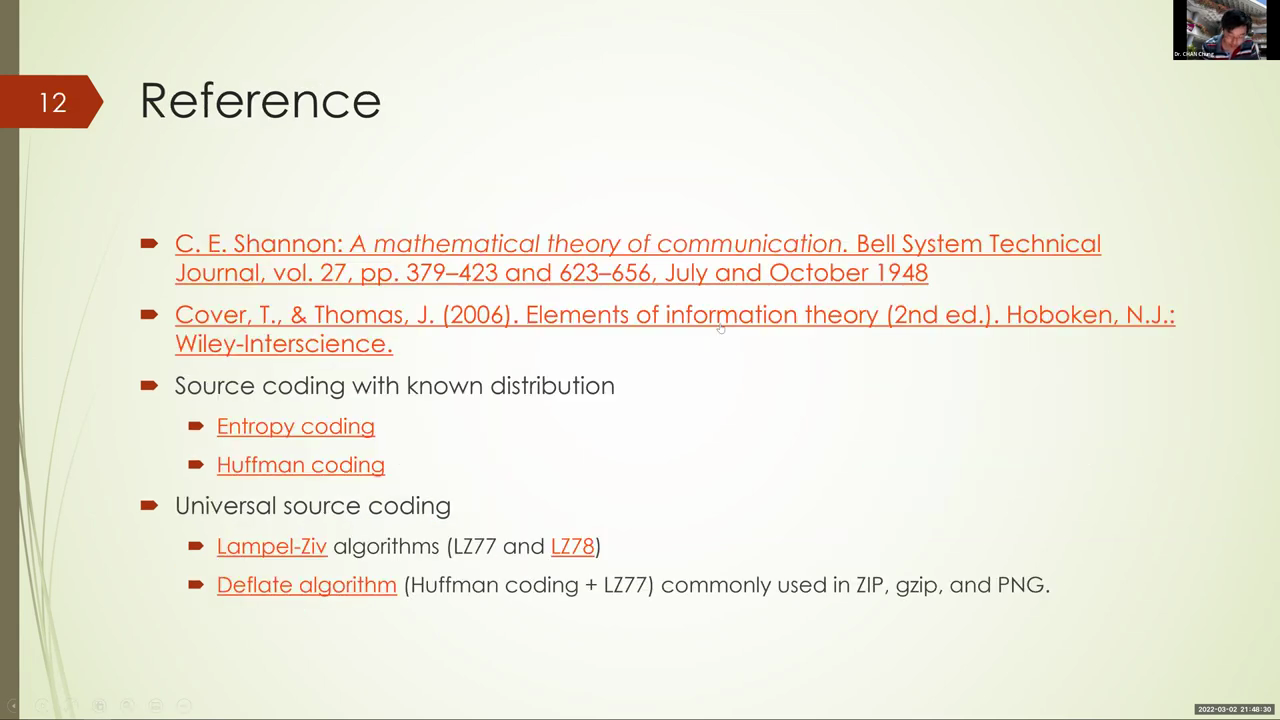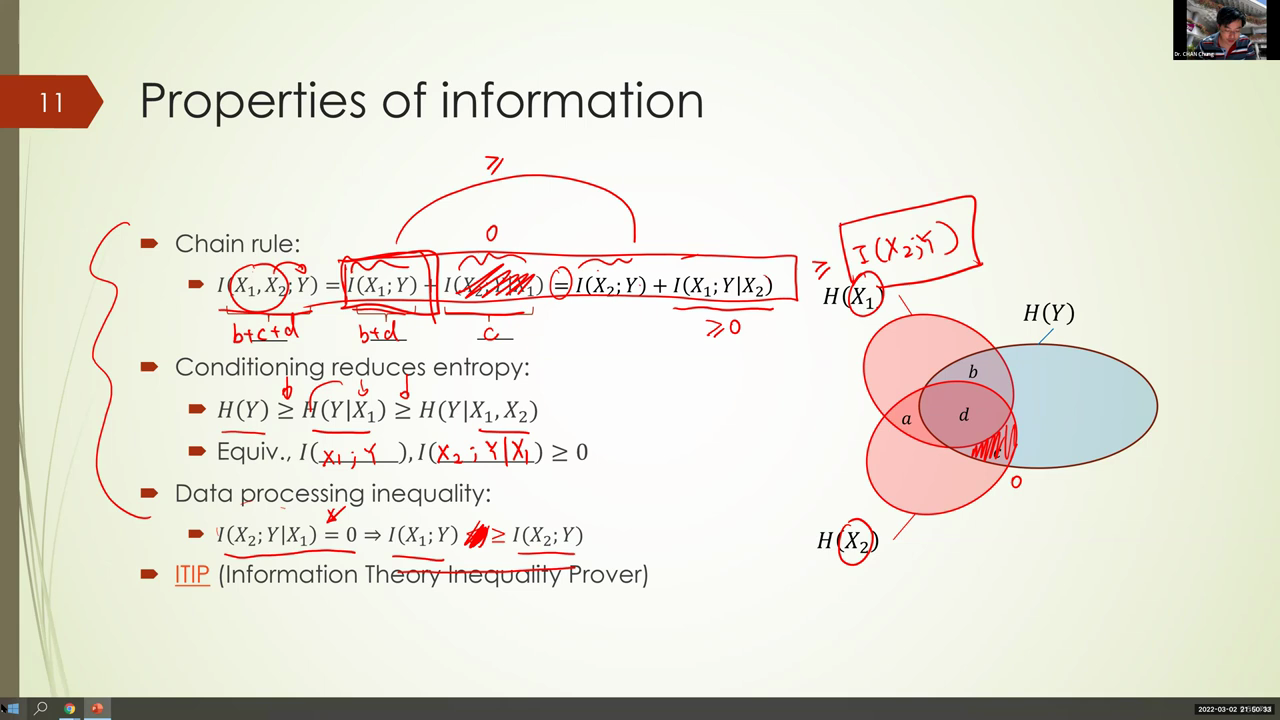Main point
Full script

Okay, this mutual information is not negative. I can further write this as bigger than the mutual information between x2 and y. Now notice, this terms goes away by the premise of data in processing inequality. So we are only left with this term on the left and this term on the right, and that is exactly the inequality we want to prove. So this is data processing in party. Okay, so indeed, there are many inequality you can prove. Some are not very obvious. I mean, what i've shown you are those obvious ones, but then there are- there can be more complicated inequalities, indeed all of them follow just from the basic ones you can show right here, indeed it you can well. A lot of the inequalities is based on the property of entropy, called as a modularity, and this is well beyond what is even for an information theory course will require. So now there is a, a program called the itip, and this will automatically prove whether certain information inequality works or not. You can try it out and see. I mean all this inequality proved using this information in quality prover. There are also inequality that are hard to prove. Indeed that cannot be proved from the sor generality of entry or from from this approval. Those are non-shannon type inequality and those are inactive still in the fd area of research. Still no, very little people. I mean we, we know actually very little about those inequality.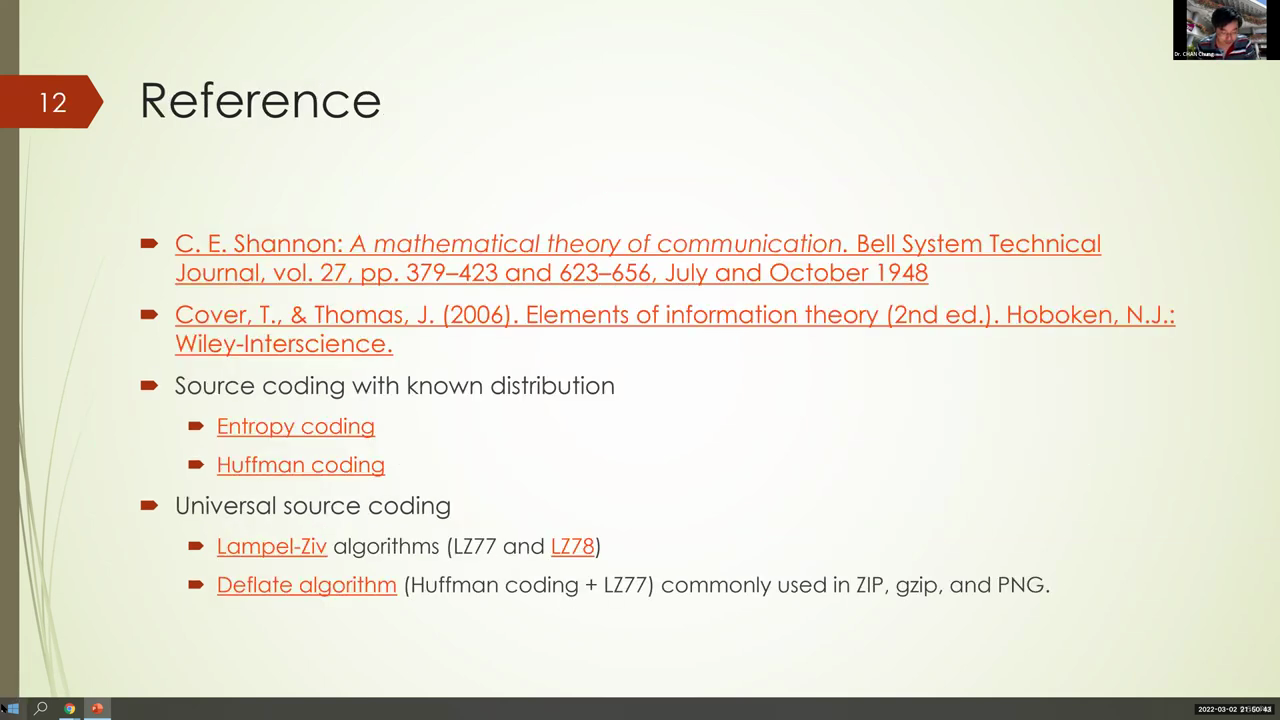Main point
Full script

Okay, with that, this complete lecture today. This lecture indeed mentioned the fundamental work of shannon half a century ago, the paper. You can read it indeed it is very long paper, many pages, but then it is very easy to read because it is written in a style that is very intuitive and convincing. Often the case the the author who need to start a new field. He has to put a lot of what may work in in terms of convincing other people to to follow his idea, and you can see a lot of very elegant idea from this paper. The other textbook i would recommend is the information tv tech books, that is, the elements of information theory. So if you're in into i mean the information theory, which i think is fundamental in data science, then i would recommend you to start with this elements of information theory. It is not about data science, though. This is more about the communication problem. Indeed, information theory has many applications. It has application to telecommunication, network coding and all that. It also have application in security problems. The source coding problem is indeed what gives a very concrete operational meaning to entropy or information. We have described, indeed, something called the entropy coding. Today there are also something called the huffman coding you can explore, and if we want to encode something without knowing the underlying probability distribution, then there's also this lamppositive algorithm, which is the sig algorithm that compress your file. Okay, of course there are. I mean right now the zip algorithm we use has additional features other than the lambda shift original amazon. Okay, and you can check the deflate algorithm and all that okay. So with that, yeah, there are a few- i mean interesting stories behind.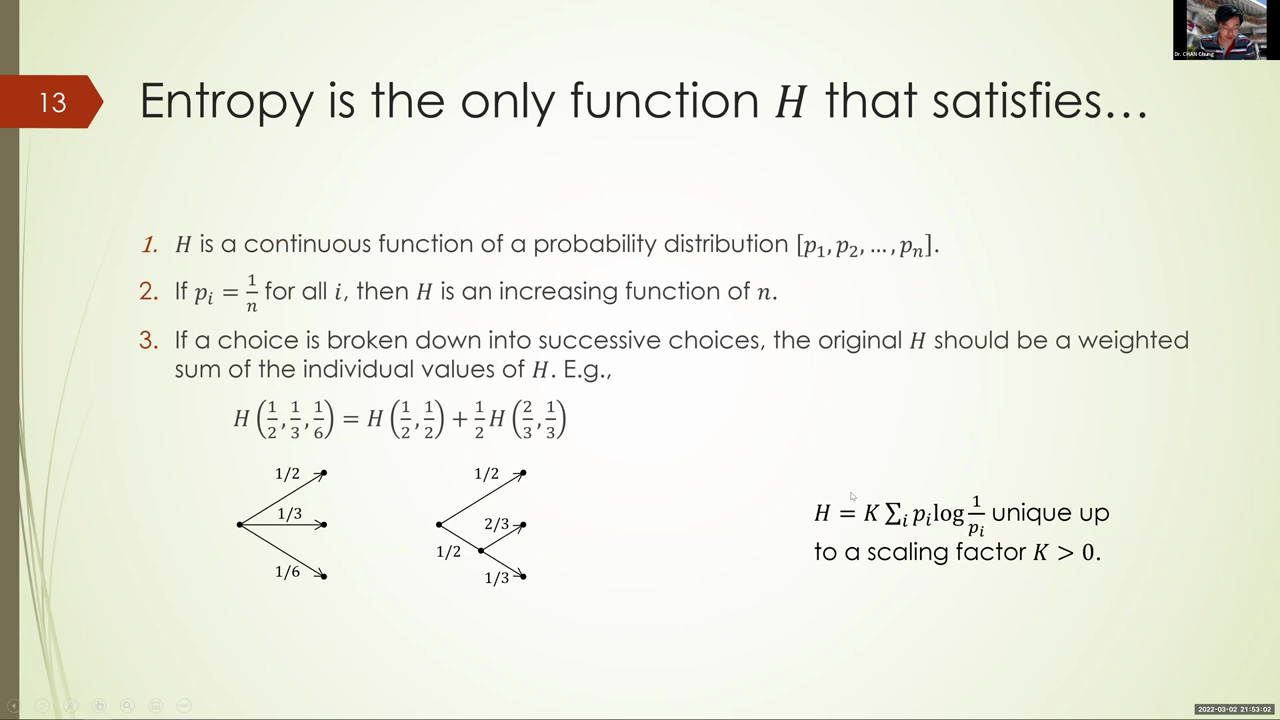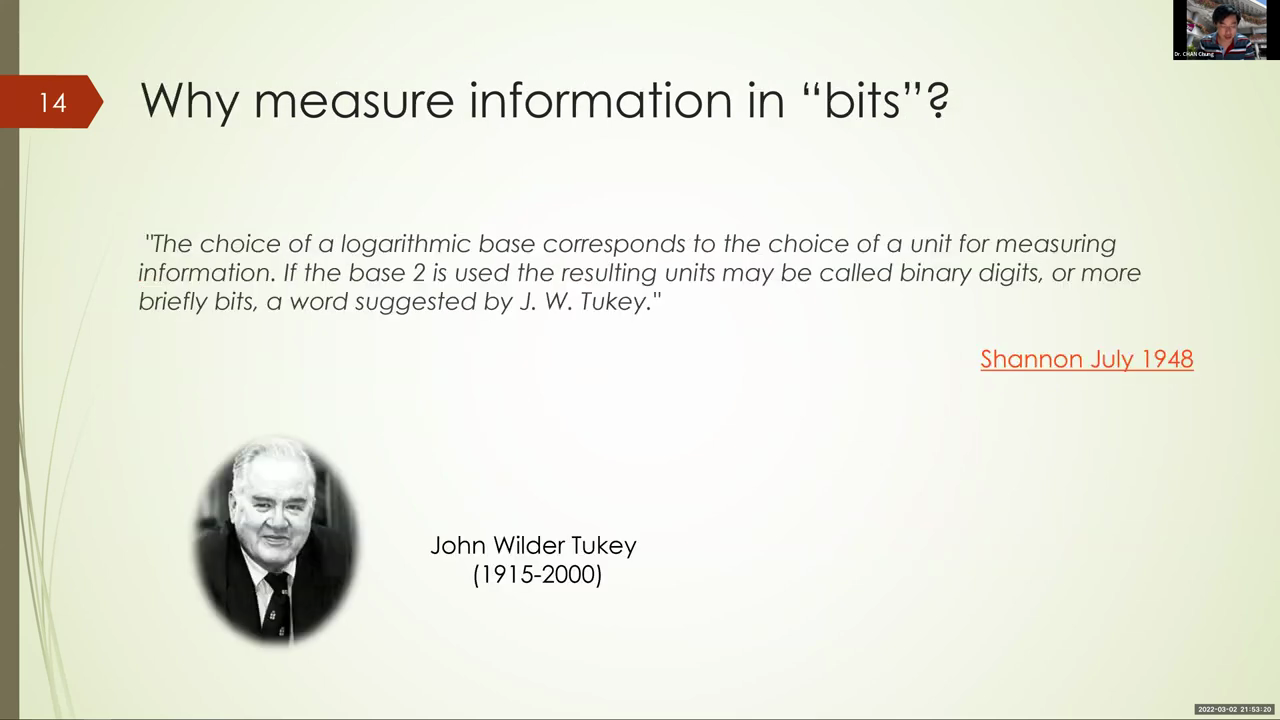Main point
Full script

You can take a look at this- that there's a fundamental features, fundamental axioms, that is satisfied by entropy. And then there's also an attribute to who. Who? Who coined the bits? Who? Who first come up with this term? This shannon is the one, is the person who used bits in the paper first, but then he attribute this name to john ritter, turkey.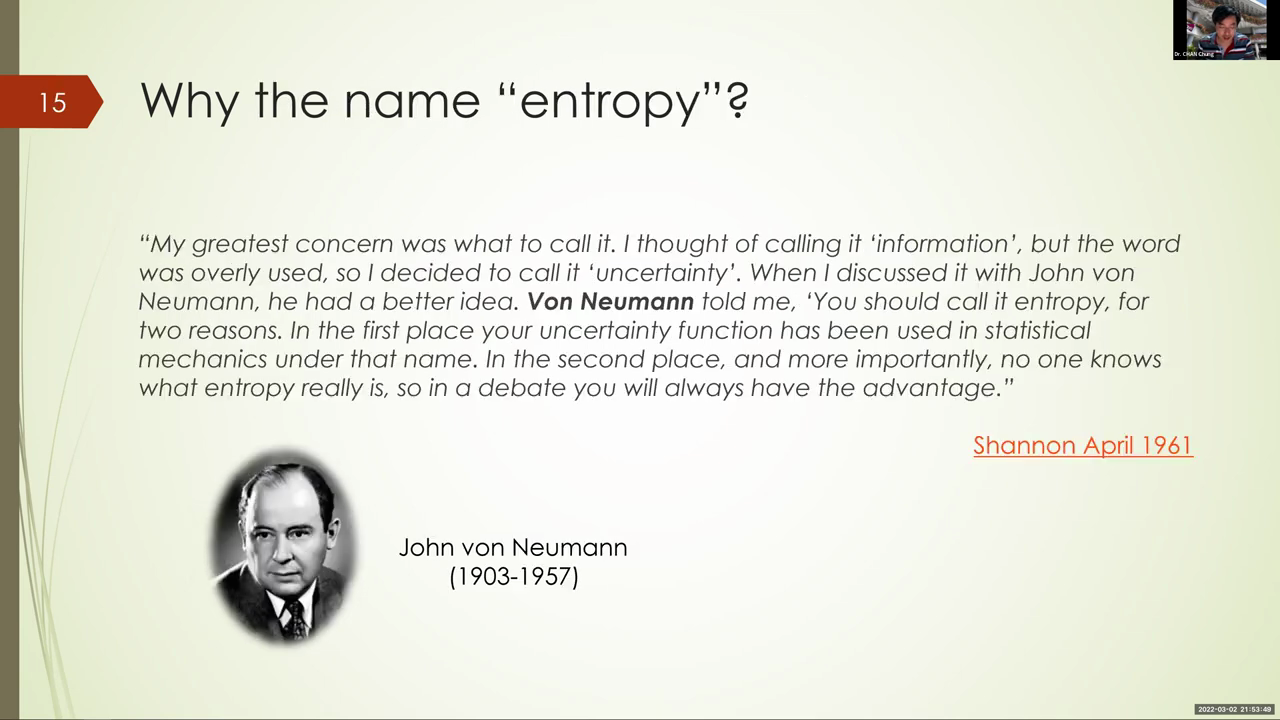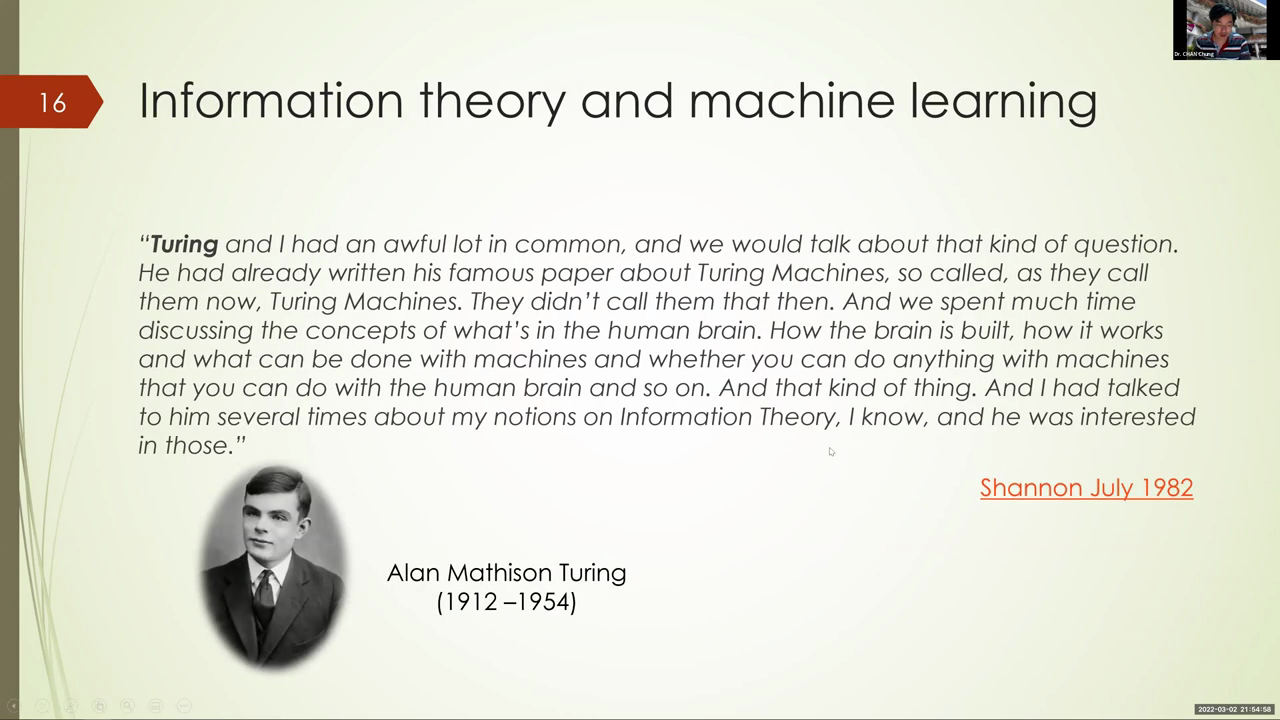Main point
Full script

And for newman, who invented this? Basically, well, who? Who actually applied the first electronic computer called aniak, to design their hydrogen bond using monte carlo simulation? He actually is the one who suggested the term entropy to shannon, so you can use: okay. So that's an interesting quote from him saying: okay in a debate. Well, what? Why choose the word entropy? Well, in the first place, your uncertainty function. Well, should be. Well, sorry, he said you should call it entropy for two reasons. In the first place, this uncertainty function has already been used in previous work, also called entropy in. The other reason is that no one know what entropy really is. So in a debate, you will always have the advantage, because when you say something that sounds very technical, then you are convincing sometimes. Okay, good, that's all. Sorry for overrunning.Main point
Full script

And now let me just mention that there are two notebooks, tutorial notebooks. They are a bit theoretical indeed, i asked you to prove something. The proof should be manageable, but yes, so it is kind of too much beyond what we require for the course. Again, i'm regarding all the tutorial notebook as optional, so so that should be okay. So you, if you don't want to do it, then that's fine, but then if you do it, then it may count towards your bonus. Now, last week tutorial has been deleted by one week, so the deadline for last week tutorial will be next week, but then the tutorial for this topic, information theory, will also be due at the same day, because the i want the the grader to be able to respond to you in the week after the lecture has been into.Main point
Full script

Well, to be in two weeks after the lecture has been released. So to avoid too much delay, this will be due on the same thing, okay. With that said, that's all for the electric today. Okay, so any questions? I'll stay behind for questions. So if you don't have questions you may need, okay. If you have questions about grading, please send us an email and indeed, lets you know. Shu is [Music] the ta who are responsible for the for the homework grading.Main point
Full script